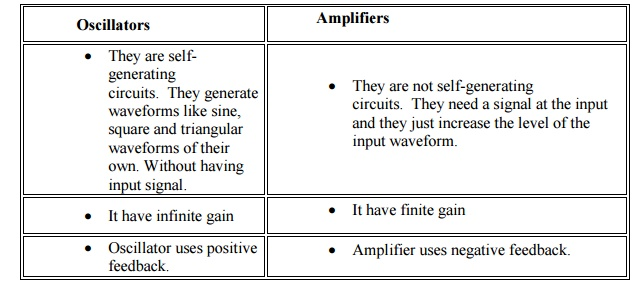Home | | Electronic Devices and Circuits | Important Short Questions and Answers: Feed Back Amplifiers and Oscillators

# Important Short Questions and Answers: Feed Back Amplifiers and Oscillators

Electronic Devices and Circuits - Feed Back Amplifiers and Oscillators - Important Short Questions and Answers: Feed Back Amplifiers and Oscillators

FEEDBACK AMPLIFIERS AND OSCILLATORS

1. What is the necessary condition for a Wien bridge oscillator circuit to have sustained oscillations?

Then for oscillations to occur in a Wien Bridge Oscillator circuit the following conditions must apply.

·                    With no input signal the Wien Bridge Oscillator produces output oscillations.

·                    The Wien Bridge Oscillator can produce a large range of frequencies.

·                    The Voltage gain of the amplifier must be at least 3.

·                    The network can be used with a Non-inverting amplifier.

·                    The input resistance of the amplifier must be high compared to R so that the RC network isnot overloaded and alter the required conditions.

·                    The output resistance of the amplifier must be low so that the effect of external loading is minimised.

2. Define piezoelectric effect.

The piezo electric Crystals exhibit a property that if a mechanical stress is applied across one face the electric potential is developed across opposite face and viceversa. This phenomenon is called piezo electric effect.

3. Differentiate oscillator from amplifier.

Oscillators Amplifiers

1. They are self-generating circuits. They generate waveforms like sine, square and triangular waveforms of their own. Without having input signal.

2. They are not self-generating circuits. They need a signal at the input and they just increase the level of the input waveform.

3. It have infinite gain

2. It have finite gain

4. Oscillator uses positive feedback.

5.  Amplifier uses negative feedback.

4. State Barkhausen criterion for sustained oscillation. What will happen to the oscillation if the magnitude of the loop gain is greater than unity?

The conditions for oscillator to produce oscillation are given by Barkhausan criterion. They are : i) The total phase shift produced by the circuit should be 360o or 0o

ii) The Magnitude of loop gain must be greater than or equal to 1 (ie)|Aβ|≥1

In practice loop gain is kept slightily greater than unity to ensure that oscillator work even if there is a slight change in the circuit parameters.

5. State any two methods of achieving sweep linearity of a time-base waveform.

·                    Exponential charging

·                    Constant current charging

·                    Miller circuit

·                    Bootstrap circuit.

6. Draw the equivalent circuit of a pulse transformer. Name the various elements in it.R1=Primary winding and source resistance

R2=Total resistance reflected to primary side =Leakage inductance

L=Magnetizing inductance

C=Total effective shunt capacitance

7. What is the function of time base circuit?

A linear time base generator produces an output waveform, which produces a portion which exhibits a linear variation of voltage or current with respect to time.

8. Define feedback. What are the types of it?

The process of combining a fraction or part of output energy back to the input is known as feedback. The different types of feedback are:

a.                         Positive feedback, b.  Negative feedback

9. What is meant by a) positive feedback b) negative feedback? Positive feedback

If feedback signal applied is in phase with the input signal and thus increases the input, it is called as positive feedback. It is also known as regenerative feedback.

Negative feedback

If the feedback signal applied to the input is out of phase with the input signal and thus signal decrease, it is called negative feedback. It is also known as degenerative feedback.

10.            What are the advantages of negative feedback?

·                                                                    It improves the stability of the circuit.

·                                                                    It improves the frequency response of the amplifier.

·                                                                    It improves the percentage of harmonic distortion.

·                                                                    It improves the signal to noise ratio (SNR).

·                                                                    It reduces the gain of the circuit.

11.            Define the feedback factor β?

It is the ratio between the feedback voltages to the output voltage of the amplifier.

β = Vf/ Vo

Where, β is a feedback factor (or) feedback ratio, Vf is the feedback voltage, Vo is the output voltage.

12. What is transresistance amplifier?

In voltage shunt feedback amplifier the sampled signal is a voltage and the feedback signal (Which is fed in shunt) is a current.

Rm = Vo / Ii (or) Vo = Rm. Ii Where, Rm = Amplifier gain.

Vo = Output voltage. Ii = Input current.

13.How does an oscillator differ from an amplifier?14.What is Barkhausan criterion?

·                                The conditions for oscillator to produce oscillation are given by Barkhausan criterion. They are:

·                                The total phase shift produced by the circuit should be 360o or 0o

·                                The Magnitude of loop gain must be greater than or equal to 1

15.            List the disadvantages of crystal Oscillator.

·  It is suitable for only low power circuits

·                                            Large amplitude of vibrations may crack the crystal.

·                                            It large in frequency is only possible replacing the crystal with another one by different frequency.

16.            Write the expression for frequency of Oscillation of a colpitts oscillator.Study Material, Lecturing Notes, Assignment, Reference, Wiki description explanation, brief detail
Electronic Devices and Circuits : Feed Back Amplifiers and Oscillators : Important Short Questions and Answers: Feed Back Amplifiers and Oscillators |